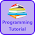# Programming Videos

## Search

### Java Program to Find HCF of Two Numbers using Recursion

Write a program to find HCF of two numbers using recursion in Java. Given two numbers, we have to write a Java code to print HCF (Highest Common Factor) of two numbers.

Before start writing a code, let's first understand what is HCF?

## What is HCF?

HCF (Highest Common Factor) of two numbers a and b is the largest positive integers that divide both the numbers (a and b).

For example - Suppose the value of a is 12 and the value of b is 15.

Factors of 12 is 1, 2, 3, 4, 6, 12

Factors of 15 is 1, 3, 5, 15

Common factors of 12 and 15 is 1, 3

Highest common factor of 12 and 15 is 3.

Program to Find HCF or GCD of two numbers using Recursion in C, C++

Recursion

## Java Program to Find HCF of Two Numbers using Recursion

```public class HCF {

public static int calculateHCF(int a, int b) {

if (a == b) {
return a;
} else if (a == 0) {
return b;
} else if (b == 0) {
return a;
} else if (a > b) {
//Recursively call
return calculateHCF(a % b, b);
} else {
//Recursively call
return calculateHCF(a, b % a);
}

}

public static void main(String[] args) {

Scanner in = new Scanner(System.in);

System.out.println("Enter first number");
int a = in.nextInt();

System.out.println("Enter second number");
int b = in.nextInt();

System.out.println("HCF of two numbers is " + calculateHCF(a, b));

}

}

```

Java program to count number of digits in a number

Java program to print factorial of a number

Programming questions on Recursion

1.1.